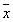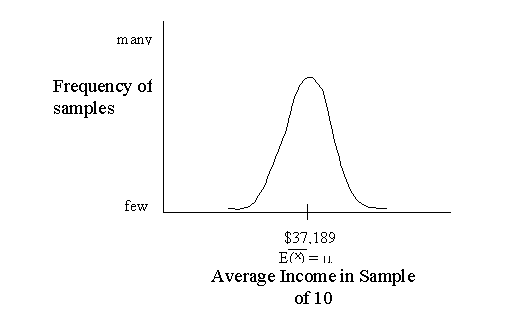Lecture 2
Sampling Distributions

Sample vs. Population
Researchers distinguish between samples and populations. A population is a large group of people to which we are interested in generalizing. Examples might be: all people residing in the U.S., all married females between ages 35 and 44, all children under age 3, all hospitals in the U.S. A sample is simply a smaller group drawn from a population. For example: 1,000 people residing in the U.S., 120 women between ages 35 and 44, 967 children under age 3, or 100 hospitals in the U.S.

A numerical summary of some kind, such as an average, has two different forms: one form is used for the sample and one form is used for the population. In Daniel's notation the average for a sample is written asand the average for the population is written as m (the Greek letter "mu"). The sample average () is a called a statistic and m is called a parameter. If you do not remember some of the other common statistics and parameters, such as the mean, median, mode, standard deviation, and variance, I suggest a review of Chapter 2 in the Daniel text.

Descriptive vs. Inferential Statistics
Most people think of descriptive statistics when they hear the word "statistics." For example, batting averages in baseball or "4 out of 5 dentists recommend…" But we are not really going to study that type of statistics in this class. We are more interested in inferential statistics, because those are the statistics that most researchers use. The difference is that inferential statistics infer something about a population, based on a smaller number of people, a sample. The whole enterprise of inferential statistics is about making numerical guesses about a population based on a sample. Descriptive statistics is merely for the purposes of describing the sample. In descriptive statistics, results are not really meant to apply to other samples or to the larger population.

Significance
Statistical significance is a particular term that researchers use. Significance refers to a kind of certainty about whether or not some result in a particular sample is a result that is likely to be true in population from which it is drawn or other samples drawn from that population. For instance, if 32.1% of the sample report having not seen a doctor in the past year, how do we know whether approximately 32% in the entire population has not seen a doctor in the past year? Or, if a sample of employees in the Portland metropolitan area have an average income of \$37,189, how do we know if this result is likely to be true if we surveyed all of the employees in the Portland metro area?

Now, one of the reasons why the sample statistic might not be a good approximation of the population parameter is that the sample was not a very good sample. That is, a small unrepresentative sample was drawn for some reason. There are several ways to draw samples for the population, some of them are good ways and some of them are not so good. The better ways will produce samples that are representative of the population (have all the same characteristics). For this class, we are not going to discuss the methodology involved in drawing samples, and we will assume that we have done a good job of that. For instance, a good way to draw a sample is to take a random sample, in which every member of the population has an equal chance of being selected for the sample.

Sampling Variability
Even if we draw a perfectly random sample, we are not very likely to get the same result in the sample as we would in the population. Just by chance we are likely to get a somewhat different set of folks in the sample, and this is called sampling variability. Let's say we could pick 10 employees from the Portland metro area perfectly by random. Let's also assume the mean income for all of Portland metro employees is \$37,189. It is very unlikely that the average income of the 10 people in our sample is going to be exactly \$37,189. We might, for instance, pick 10 employees from fast food restaurants completely by chance. Their average income might be only about \$10,000. Or we might get a sample of lawyers, doctors, and cooperate executives by chance, and their average income might be \$157,000. It's probably pretty unlikely we would draw either of these samples because they are extreme. It should be more likely that we would get a result that is close to \$30,000 or \$40,000.

Sampling Distribution
So, in this example it is more likely we will get sample means that are somewhere around \$37,000. If we took a large number of samples of 10 employees and calculated the average for each, there should be a large number of sample averages around 37,000, but we would also get a few weird ones that are far from that number. Now, if we were to take these sample averages and plot them in a frequency diagram, it might look something like this:In the above figure, most of the samples are around the middle. The middle value, which is called the expected value of the mean (represented by E() ), is equal to the mean of all the sample means (i.e., take the mean of each sample and then take the mean of all those means). It just so happens that the expected mean is equal to the population mean. That is, if we collected an infinite number of samples and calculated the mean of each, and then calculated the mean of all those sample means, it would equal the mean of the entire population.

The figure above represents the sampling distribution of the mean. The sampling distribution is like other distributions because it has a mean (which is called the expected mean) and a standard deviation (called the standard error). Because we calculated the mean in each sample, the standard error is called the standard error of the mean. We could have calculated other statistics in each sample, like the median or the standard deviation, in which case we would have the standard error of the median or the standard error of the standard deviation. (It turns out that neither of the latter are used very often). Every statistic has a sampling distribution though. A statistic is unbiased if it's sampling distribution mean is equal to the population mean. The sample mean,, is unbiased.

This all probably sounds pretty hypothetical (and hopefully familiar), but it is the basis of all of inferential statistics. Inferential statistics, statistical tests, and statistical significance are all based on guesses about sampling variability. If we know about how the mean fluctuates from sample to sample due to chance, we can use that information to make inferences about the population.